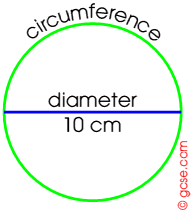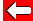# Approximation

The special number pi is about 3, but using 3 in calculations is not very accurate. Using 3·14 instead is more useful, whilst scientific calculators are usually pre-programmed with pi as 3·14159265359

Let's use these different approximations of pi to calculate the circumference of a circle, diameter d=10 cm.The circumference c is given by: c = pi × d. 1. c = pi × d = 3 × 10 = 30 cm 2. c = pi × d = 3·14 × 10 = 31·4 cm 3. c = pi × d = 3·14159265359 × 10 = 31·4159265359 cm (1) is about right; (2) is quite accurate (nearest millimetre); whereas (3) is excessively accurate: do we need an answer to the nearest billionth of a cm?! Vital Question: how accurate was the 10 cm diameter? Did we assume it was perfect, or to the nearest cm or mm?Go back a pageMaths MenuGo to next page